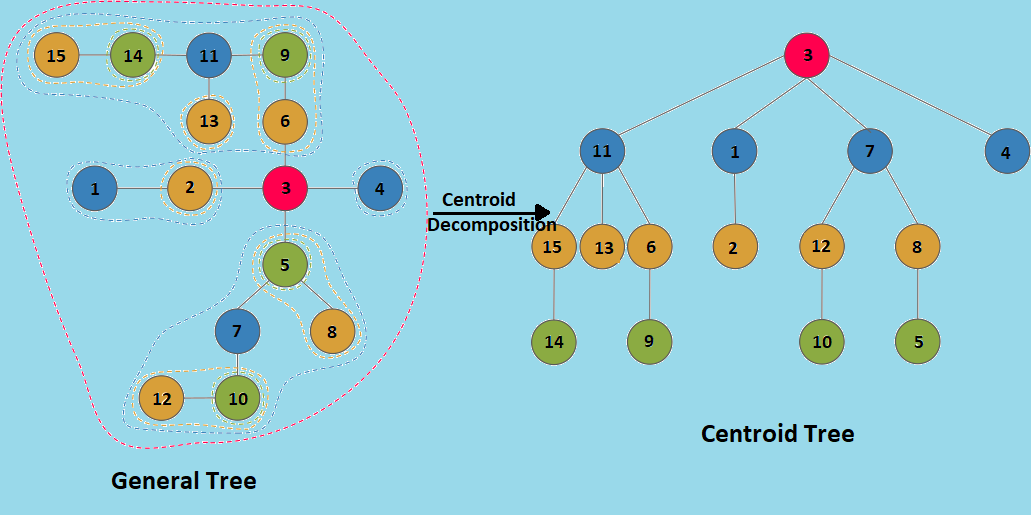×

Search anything:

# Centroid Decomposition of Tree

#### Algorithms divide and conquer depth first search Graph Algorithms centroid decompositionGet this book -> Problems on Array: For Interviews and Competitive Programming

Centroid Decomposition is a divide and conquer technique which is used on trees.
The centroid decomposition of a tree is another tree defined recursively as:

• Its root is the centroid of the original tree.
• Its children are the centroid of each tree resulting from the removal of the root(centroid) from the original tree.

Centroid of a Tree
Given a tree with N nodes, a centroid is a node whose removal splits the given tree into a forest of trees, where each of the resulting tree contains no more than N/2 nodes.# Algorithm

Finding the centroid of a tree
One way to find the centroid is to pick an arbitrary root, then run a depth-first search computing the size of each subtree, and then move starting from root to the largest subtree until we reach a vertex where no subtree has size greater than N/2. This vertex would be the centroid of the tree i.e. Centroid is a node v such that,

```maximum(N - S(v), S(u1, S(u2, .. S(um) <= N/2
where ui is ith child of v,
and S(u) is the size(number of nodes in tree) of subtree rooted at u.
```

Algorithm

1. Select arbitrary node v
2. Start a DFS from v, and setup subtree sizes
3. Re-position to node v (or start at any arbitrary v that belongs to the tree)
4. Check mathematical condition of centroid for v
1. If condition passed, return current node as centroid
2. Else move to adjacent node with `greatest` subtree size, and back to step 4.

Decomposing the Tree to get the new "Centroid Tree"
On removing the centroid, the original given tree decomposes into a number of different trees, each having no of nodes < N/2 . We make this centroid the root of our centroid tree and then recursively decompose each of the new trees formed and attach their centroids as children to our root. Thus , a new centroid tree is formed from the original tree.

Algorithm

1. Make the centroid as the root of a new tree (which is centroid tree).
2. Recursively decompose the trees in the resulting forest.
3. Make the centroids of these trees as children of the centroid which last split them.

example: For the following tree:Number of children of any node of a tree can be O(logN) in the worst case. So in each find_centroid operation which takes O(N) time in worst case, we divide the tree into forest of O(logN) subtrees, so we recur at most O(logN) times. So overall centroid decomposition of a tree takes O(N logN) time in the worst case.

# Implementation

Code in Python3

``````import sys

class Tree:
def __init__(self, n):
self.size = n + 1
self.cur_size = 0
self.tree = [[] for _ in range(self.size)]
self.iscentroid = [False] * self.size
self.ctree = [[] for _ in range(self.size)]

def dfs(self, src, visited, subtree):
visited[src] = True
subtree[src] = 1
self.cur_size += 1

def findCentroid(self, src, visited, subtree):
iscentroid = True
visited[src] = True
heavy_node = 0
iscentroid = False

if heavy_node == 0 or subtree[adj] > subtree[heavy_node]:

if iscentroid and self.cur_size - subtree[src] <= self.cur_size//2:
return src
else:
return self.findCentroid(heavy_node, visited, subtree)

def findCentroidUtil(self, src):
visited = [False] * self.size
subtree =  * self.size
self.cur_size = 0
self.dfs(src, visited, subtree)
for i in range(self.size):
visited[i] = False
centroid = self.findCentroid(src, visited, subtree)
self.iscentroid[centroid] = True
return centroid

def decomposeTree(self, root):
centroid_tree = self.findCentroidUtil(root)
print(centroid_tree, end=' ')
self.ctree[centroid_tree].append(centroid_subtree)
self.ctree[centroid_subtree].append(centroid_tree)

return centroid_tree

self.tree[src].append(dest)
self.tree[dest].append(src)

if __name__ == '__main__':
tree = Tree(15)
# A tree with 15 nodes

print("DFS traversal of generated Centroid tree is:")
tree.decomposeTree(1)
``````

Output

``````DFS traversal of generated Centroid tree is:
3 2 1 4 7 5 8 10 12 11 9 6 13 14 15
``````

# Complexity

Time Complexity

• DFS of tree takes O(N) time.
• Find_Centroid of tree takes O(N) time.
• Centroid Decomposition of tree takes overall O(N logN) time.

Space Complexity

• DFS of tree takes O(N) space.
• Adjacency list of tree takes O(N2) auxiliary space in worst case.
• Overall time space used by Centroid Decomposition algorithm is O(N2)

# Application

Centroid Decomposition of tree is a vastly used divide and conquer technique in competitive programming. Here is a list of problems where we use centroid decomposition to solve the problem: